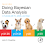## Tuesday, August 7, 2012

### Metropolis Algorithm: Discrete Position Probabilities

I was asked by a reader how I created Figure 7.2 of the book, reproduced at right, which shows the progression of discrete position probabilities at each step in a simple Metropolis algorithm. I couldn't find the original program, so I made a new one, reported here, with an additional example that illustrates a bimodal target distribution.

The main point is mentioned in the middle of p. 128: "At every time step, we multiply the current position probability vector w by the transition probability matrix T to get the position probabilities for the next time step. We keep multiplying by T over and over again to derive the long-run position probabilities. This process is exactly what generated the graphs in Figure 7.2."

The code consists of two main parts. First, build the transition matrix. Second, specify an initial position vector and repeatedly multiply by the transition matrix. Here is the code:

# For Figure 7.2, p. 123 of Doing Bayesian Data Analysis.
fileNameRoot = "DBDAfigure7pt2"

# Specify the target probability distribution.
pTargetName = "Linear" # for filename of saved graph
nSlots = 7
pTarget = 1:nSlots
pTarget = pTarget/sum(pTarget)

# Uncomment following lines for different example
#pTargetName = "Bimodal" # for filename of saved graph
#pTarget = c(1,2,2,1,3,3,1)
#nSlots = length(pTarget)
#pTarget = pTarget/sum(pTarget)

# Construct the matrix of proposal probabilities.
# Row is from position, column is to position.
proposalMatrix = matrix( 0 , nrow=nSlots , ncol=nSlots )
for ( fromIdx in 1:nSlots ) {
for ( toIdx in 1:nSlots ) {
if ( toIdx == fromIdx-1 ) { proposalMatrix[fromIdx,toIdx] = 0.5 }
if ( toIdx == fromIdx+1 ) { proposalMatrix[fromIdx,toIdx] = 0.5 }
}
}

# Construct the matrix of acceptance probabilities.
# Row is from position, column is to position.
acceptMatrix = matrix( 0 , nrow=nSlots , ncol=nSlots )
for ( fromIdx in 1:nSlots ) {
for ( toIdx in 1:nSlots ) {
acceptMatrix[fromIdx,toIdx] = min( pTarget[toIdx]/pTarget[fromIdx] , 1 )
}
}

# Compute the matrix of overall move probabilities:
moveMatrix = proposalMatrix * acceptMatrix

# Compute the transition matrix, including the probability of staying in place:
transitionMatrix = moveMatrix
for ( diagIdx in 1:nSlots ) {
transitionMatrix[diagIdx,diagIdx] = 1.0 - sum(moveMatrix[diagIdx,])
}
show( transitionMatrix )

# Specify starting position vector:
positionVec = rep(0,nSlots)
positionVec[round(nSlots/2)] = 1.0

# Loop through time steps and display position probabilities:
windows(height=7,width=7) # change to x11 for non-WindowsOS
layout( matrix(1:16,nrow=4) )
par( mar = c( 2.8 , 2.8 , 1.0 , 0.5 ) , mgp = c( 1.4 , 0.5 , 0 ) )
for ( timeIdx in 1:15 ) {
# Display position probability:
plot( 1:nSlots , positionVec , xlab="Position" , ylab="Probability" ,
type="h" , lwd=5 , col="skyblue" )
text( 1 , max(positionVec) , bquote(t==.(timeIdx)) , adj=c(0,1) )
# Update next position probability:
positionVec = positionVec %*% transitionMatrix
}
# Plot target distribution
plot( 1:nSlots , pTarget , xlab="Position" , ylab="Probability" ,
type="h" , lwd=5 , col="skyblue" )
text( 1 , max(positionVec) , bquote(target) , adj=c(0,1) )

savePlot( filename=paste(fileNameRoot,pTargetName,sep="") , type="jpg" )

For the linearly increasing target distribution, the transition matrix looks like this:
     [,1]      [,2]      [,3]  [,4]      [,5]       [,6]      [,7]
[1,] 0.50 0.5000000 0.0000000 0.000 0.0000000 0.00000000 0.0000000
[2,] 0.25 0.2500000 0.5000000 0.000 0.0000000 0.00000000 0.0000000
[3,] 0.00 0.3333333 0.1666667 0.500 0.0000000 0.00000000 0.0000000
[4,] 0.00 0.0000000 0.3750000 0.125 0.5000000 0.00000000 0.0000000
[5,] 0.00 0.0000000 0.0000000 0.400 0.1000000 0.50000000 0.0000000
[6,] 0.00 0.0000000 0.0000000 0.000 0.4166667 0.08333333 0.5000000
[7,] 0.00 0.0000000 0.0000000 0.000 0.0000000 0.42857143 0.5714286
The row is the "from" position and the column is the "to" position. For example, the probability of moving from position 4 to position 3 is 0.375.

The resulting graph looks like this:

If you uncomment the lines that specify a bimodal target, the resulting graph looks like this:

1.2.